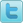Item# STP-lynn
\$119.95
Sizes::## Product Description

Length of skirt approximately 36"

Waist:

2 = 26"

4 = 28"

6 = 30"

8 = 32"

10= 34"

12= 36"

14= 38"

16= 40"

12=36"